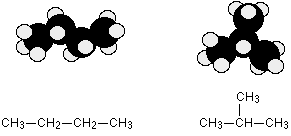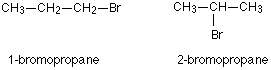# 1.6: Structural Isomerism in Organic Molecules

$$\newcommand{\vecs}{\overset { \rightharpoonup} {\mathbf{#1}} }$$ $$\newcommand{\vecd}{\overset{-\!-\!\rightharpoonup}{\vphantom{a}\smash {#1}}}$$$$\newcommand{\id}{\mathrm{id}}$$ $$\newcommand{\Span}{\mathrm{span}}$$ $$\newcommand{\kernel}{\mathrm{null}\,}$$ $$\newcommand{\range}{\mathrm{range}\,}$$ $$\newcommand{\RealPart}{\mathrm{Re}}$$ $$\newcommand{\ImaginaryPart}{\mathrm{Im}}$$ $$\newcommand{\Argument}{\mathrm{Arg}}$$ $$\newcommand{\norm}{\| #1 \|}$$ $$\newcommand{\inner}{\langle #1, #2 \rangle}$$ $$\newcommand{\Span}{\mathrm{span}}$$ $$\newcommand{\id}{\mathrm{id}}$$ $$\newcommand{\Span}{\mathrm{span}}$$ $$\newcommand{\kernel}{\mathrm{null}\,}$$ $$\newcommand{\range}{\mathrm{range}\,}$$ $$\newcommand{\RealPart}{\mathrm{Re}}$$ $$\newcommand{\ImaginaryPart}{\mathrm{Im}}$$ $$\newcommand{\Argument}{\mathrm{Arg}}$$ $$\newcommand{\norm}{\| #1 \|}$$ $$\newcommand{\inner}{\langle #1, #2 \rangle}$$ $$\newcommand{\Span}{\mathrm{span}}$$$$\newcommand{\AA}{\unicode[.8,0]{x212B}}$$

## What is structural isomerism?

Organic compounds with the same formula can have different structures. For example, two alkanes have the formula C4H10: They are called n-butane and 2-methylpropane (or isobutane), and have the following Lewis structures:Figure $$\PageIndex{1}$$: Structural isomers of C4H10

The compounds n-butane and 2-methylpropane are structural isomers (the term constitutional isomers is also commonly used). Constitutional isomers have the same molecular formula but different spatial arrangements of the atoms in their molecules. The n-butane molecule contains an unbranched chain, meaning that no carbon atom is bonded to more than two other carbon atoms. We use the term normal, or the prefix n, to refer to a chain of carbon atoms without branching. The compound 2–methylpropane has a branched chain (the carbon atom in the center of the Lewis structure is bonded to three other carbon atoms)

Identifying isomers from Lewis structures is not as easy as it looks. Lewis structures that look different may actually represent the same isomers. For example, the three structures in Figure $$\PageIndex{2}$$ all represent the same molecule, n-butane, and hence are not different isomers. They are identical because each contains an unbranched chain of four carbon atoms.Figure $$\PageIndex{2}$$: These three representations of the structure of n-butane are not isomers because they all contain the same arrangement of atoms and bonds.

There are also endless other possible ways that this molecule could twist itself. There is completely free rotation around all the carbon-carbon single bonds. If you had a model of a molecule in front of you, you would have to take it to pieces and rebuild it if you wanted to make an isomer of that molecule. If you can make an apparently different molecule just by rotating single bonds, it's not different - it's still the same molecule.

In structural isomerism, the atoms are bonded in a different arrangement. This is easier to see with specific examples. What follows looks at some of the ways that structural isomers can arise.

## Chain Isomerism

These isomers arise because of the possibility of branching in carbon chains. For example, there are two isomers of butane, $$C_4H_{10}$$. In one of them, the carbon atoms lie in a "straight chain" whereas in the other the chain is branched.##### Example $$\PageIndex{1}$$: Chain Isomers in Pentane

Pentane, C5H12, has three chain isomers. If you think you can find any others, they are simply twisted versions of the ones below. If in doubt make some models.## Position isomerism

In position isomerism, the basic carbon skeleton remains unchanged, but important groups are moved around on that skeleton.

##### Example $$\PageIndex{2}$$: Positional Isomers in C5H12

For example, there are two structural isomers with the molecular formula C3H7Br. In one of them the bromine atom is on the end of the chain, whereas in the other it's attached in the middle.If you made a model, there is no way that you could twist one molecule to turn it into the other one. You would have to break the bromine off the end and re-attach it in the middle. At the same time, you would have to move a hydrogen from the middle to the end.

Another similar example occurs in alcohols such as $$C_4H_9OH$$These are the only two possibilities provided you keep to a four carbon chain, but there is no reason why you should do that. You can easily have a mixture of chain isomerism and position isomerism - you aren't restricted to one or the other.

So two other isomers of butanol are:You can also get position isomers on rings. Consider the molecular formula $$C_7H_7Cl$$. There are four different isomers you could make depending on the position of the chlorine atom. In one case it is attached to the side-group carbon atom, and then there are three other possible positions it could have around the ring - next to the $$CH_3$$ group, next-but-one to the $$CH_3$$ group, or opposite the $$CH_3$$ group.## Functional group isomerism

In this variety of structural isomerism, the isomers contain different functional groups - that is, they belong to different families of compounds (different homologous series).

##### Example $$\PageIndex{3}$$: Isomers in C3H6O

A molecular formula $$C_3H_6O$$ could be either propanal (an aldehyde) or propanone (a ketone).There are other possibilities as well for this same molecular formula - for example, you could have a carbon-carbon double bond (an alkene) and an -OH group (an alcohol) in the same molecule.Another common example is illustrated by the molecular formula $$C_3H_6O_2$$. Amongst the several structural isomers of this are propanoic acid (a carboxylic acid) and methyl ethanoate (an ester).## Contributors

Jim Clark (Chemguide.co.uk)

This page titled 1.6: Structural Isomerism in Organic Molecules is shared under a CC BY-NC 4.0 license and was authored, remixed, and/or curated by Jim Clark.